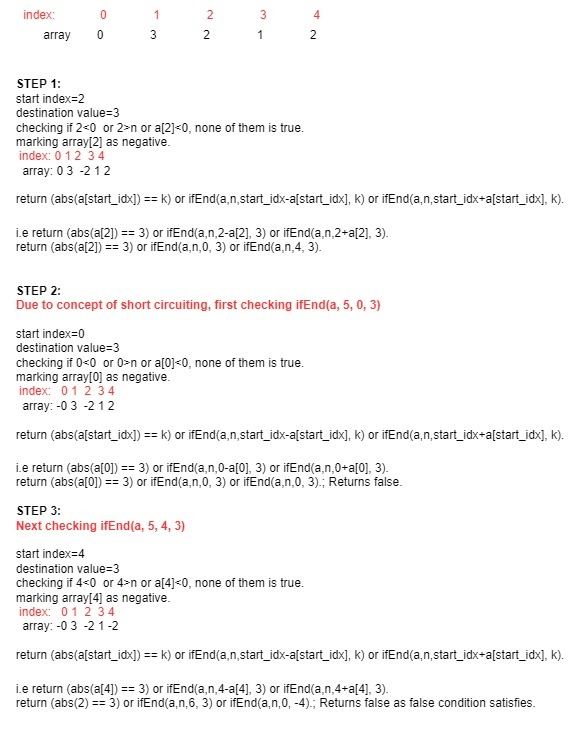# Check if it is possible to reach the index with value K when the start index is given.

## Introduction

In this blog, we will discuss a recursion problem that has been asked frequently in Interviews.

### Problem Statement

The problem is to check if it is possible to reach the index with value K when the start index is given.

We are given N numbers in an array and a starting position, and we are asked to check if an index with value k can be reached from a given position on a condition that we can only move either (current index + array[current index]) or (current index - array[current index]).

### Sample Example

Say 5 elements in an array given are: 0, 3, 2, 1, 2

And the start index given is 2.

And we need to reach 3

We must check if we can reach the destination starting from position 2, following all the given conditions.

## Approach

The approach to check if it is possible to reach the index with value K when the start index is given, would be straightforward if we follow the recursive method.

Time Complexity = O(n)

If the start index is negative or greater than the size of the array or element present at the start index is negative, we definitely can't reach the end.

If we have seen the element, then mark it visited by making the element negative.

Use recursion to change position and check if now we can get to the destination.

Solving the above given example,### PseudoCode

Algorithm

___________________________________________________________________

procedure ifEnd(int a[], int n, int start_idx, int k):

___________________________________________________________________

1.   If start_idx<0 or start_idx>n or a[start_idx]<0

return false;

2.   a[start_idx]=a[start_idx]*-1

3.   return (abs(a[start_idx]) == k) or ifEnd(a,n,start_idx-a[start_idx], k) or ifEnd(a,n,start_idx+a[start_idx], k).

4.   Declare main function, give user input and return answer.

end procedure

___________________________________________________________________

## Implementation in C++

``````//Code to Check if it is possible to reach the index with value K when the start index is given.

#include <iostream>

using namespace std;

bool ifEnd(int a[], int n, int start_idx, int k){

// If start index is negative or greater than size of array or element present at the start index is negative then definitely we can't reach the end.
if(start_idx<0 || start_idx>n || a[start_idx]<0){
return false;
}

// If we have seen the element, then mark it visited by making the element negative.
a[start_idx]=a[start_idx]*-1;

// Using recursion to change position and checking if now we can reach the destination.
return (abs(a[start_idx]) == k) || ifEnd(a,n,start_idx-a[start_idx], k) || ifEnd(a,n,start_idx+a[start_idx], k);
}

int main(){
int n;
cin>>n;
int a[n];

//enter start index
int s;
cin>>s;

//enter value of the destination position.
int k;
cin>>k;

//enter elements of array.
for(int i=0; i<n; i++){
cin>>a[i];
}

bool result=ifEnd(a,n,s, k);
if(result==1)
cout<<"yes";
else
cout<<"No";
}``````

Output:

``````Sample Input:
5
0 3 2 1 2
2
3
Sample Output:
No``````

### Complexity Analysis

Time Complexity: O(n).

Analysing Time Complexity:

In the worst-case, all elements are traversed once.

∴O(n).

Space complexity: O(n). An array of size n is required.

## FAQs

1. What is abs() function in c++? How much space does a recursive algorithm take?
abs() function is used to calculate the absolute value of a number. Space complexity in recursive algorithm directly depends upon the number of function calls and the depth of the recursion tree.

2. What is recursion?
The calling function itself, again and again, is referred to as recursion. To know more about recursion, click here.

3. Which data structure is used in recursion? How is it used?
Stack is used in recursion. When a recursive function is called, it gets stored on the top of the file (like a pile of files) and uses the last in first out technique.

## Key Takeaways

This article taught us how to Check if it is possible to reach the index with value K when the start index is given by approaching the problem using recursion. We discussed its implementation using illustrations, pseudocode, and then proper code.

We hope you could take away critical techniques like analyzing problems by walking over the execution of the examples and finding out the recursive pattern followed.

Now, we recommend you practice problem sets based on recursion to master your fundamentals. You can get a wide range of questions similar to this on CodeStudio